# R S Aggarwal Solutions for Class 11 Maths Chapter 29 Mathematical Reasoning Exercise 29A

The R S Aggarwal Class 11 solutions for the chapter Mathematical Reasoning exercise 29A is given here. This exercise is based on to check whether the given sentences are true statements or false statements. Sentences, a group of words which makes complete sense. Sentences are classified as declarative, imperative, exclamatory and interrogative. The comprehensive RS Aggarwal Class 11 Solutions to the exercise questions can be checked from the following link.

## Download PDF of R S Aggarwal Solutions for Class 11 Maths Chapter 29 Mathematical Reasoning Exercise 29A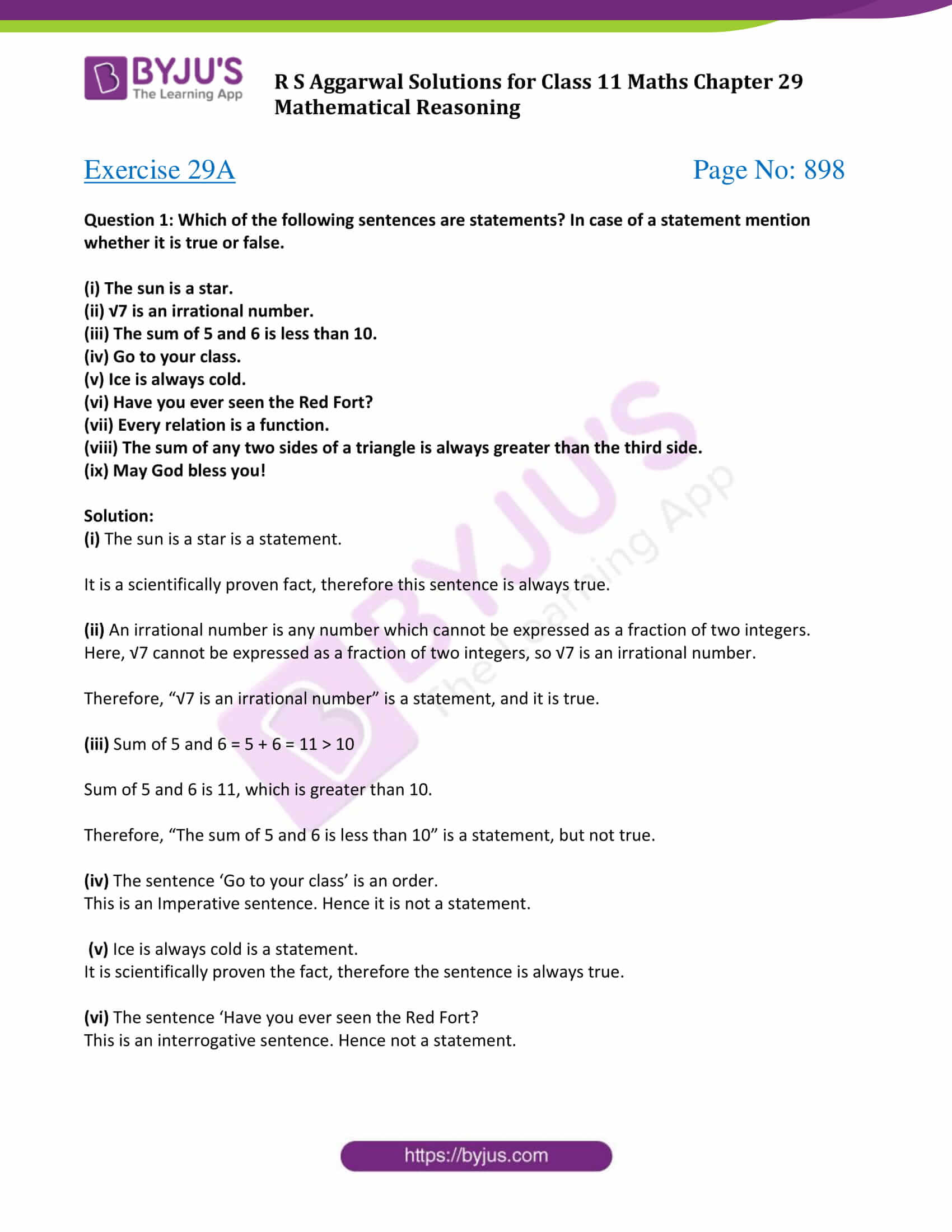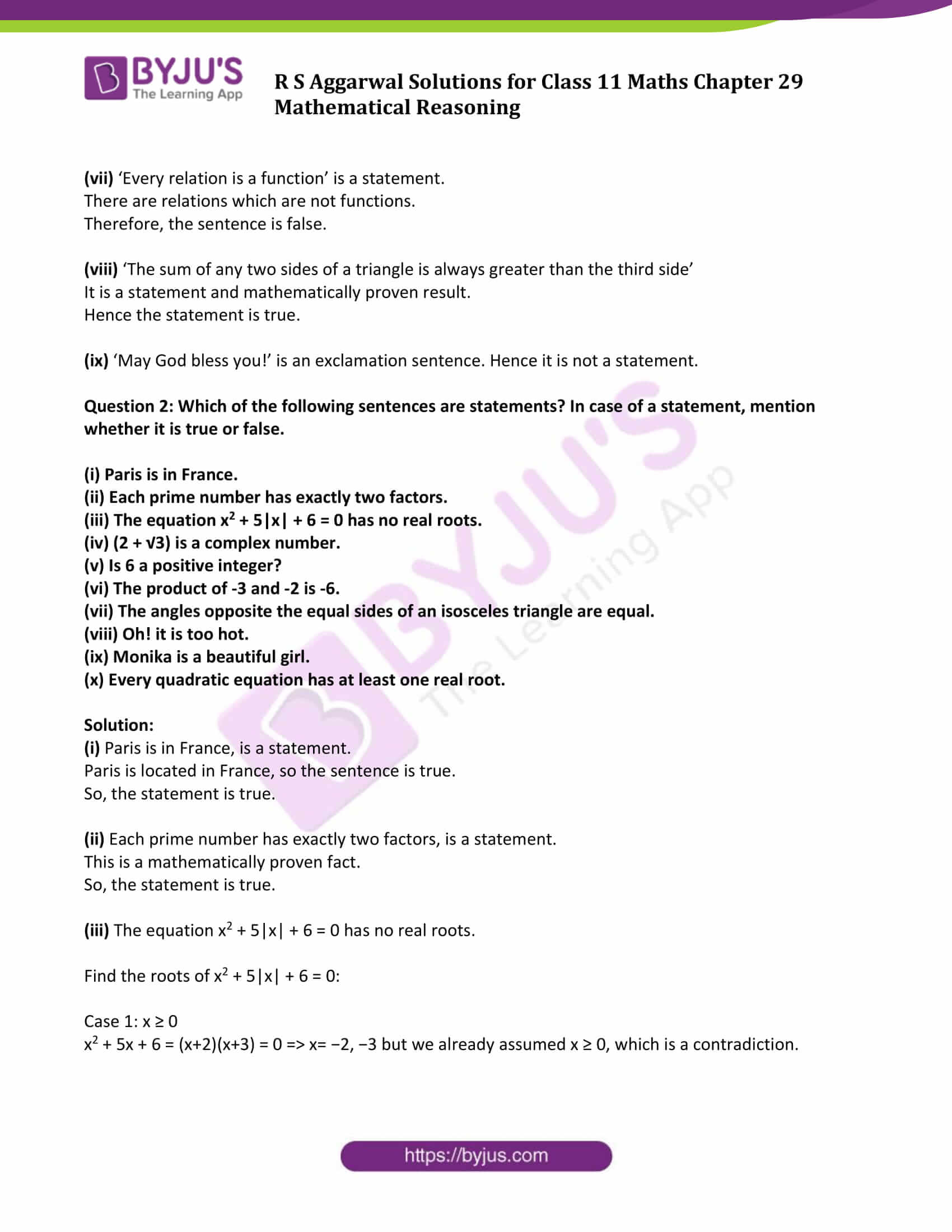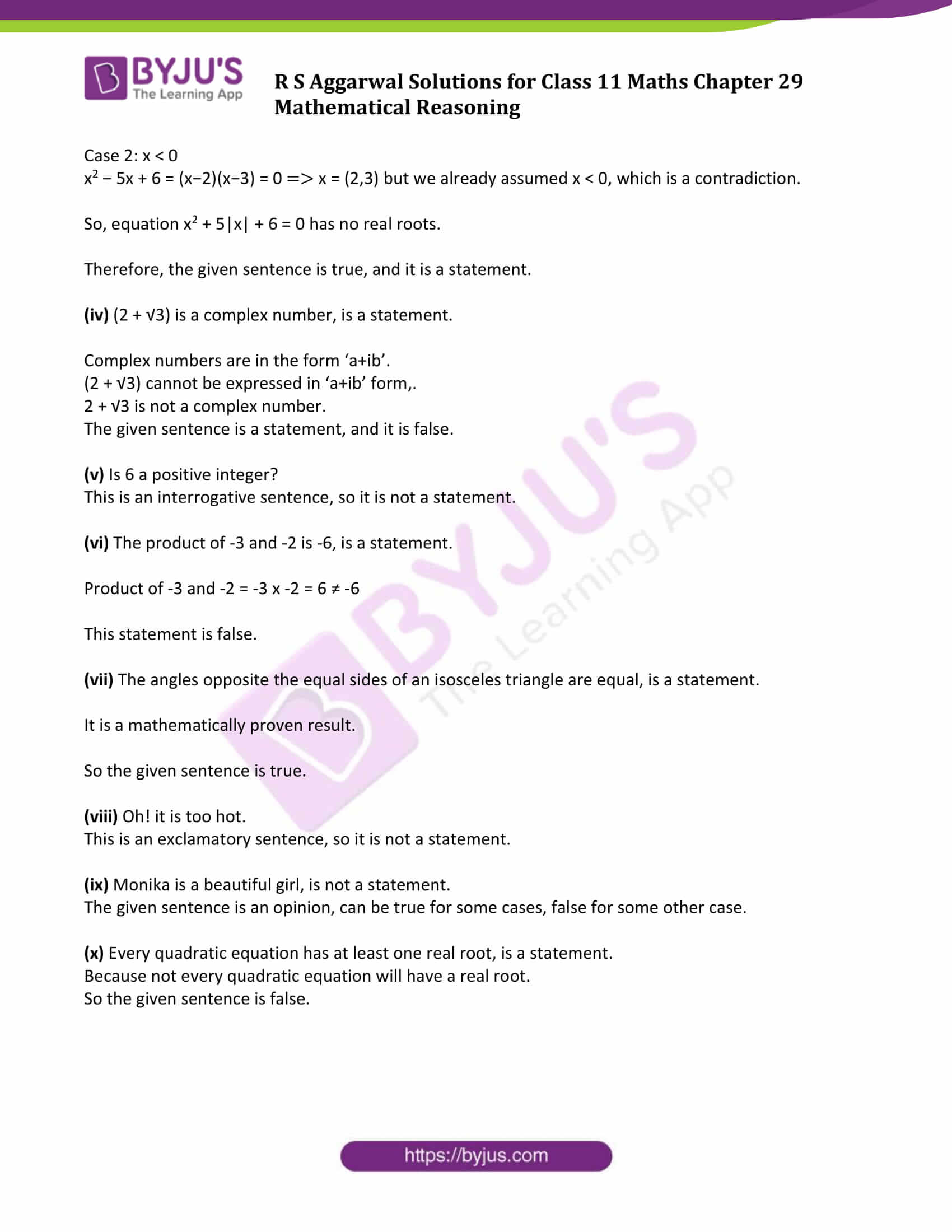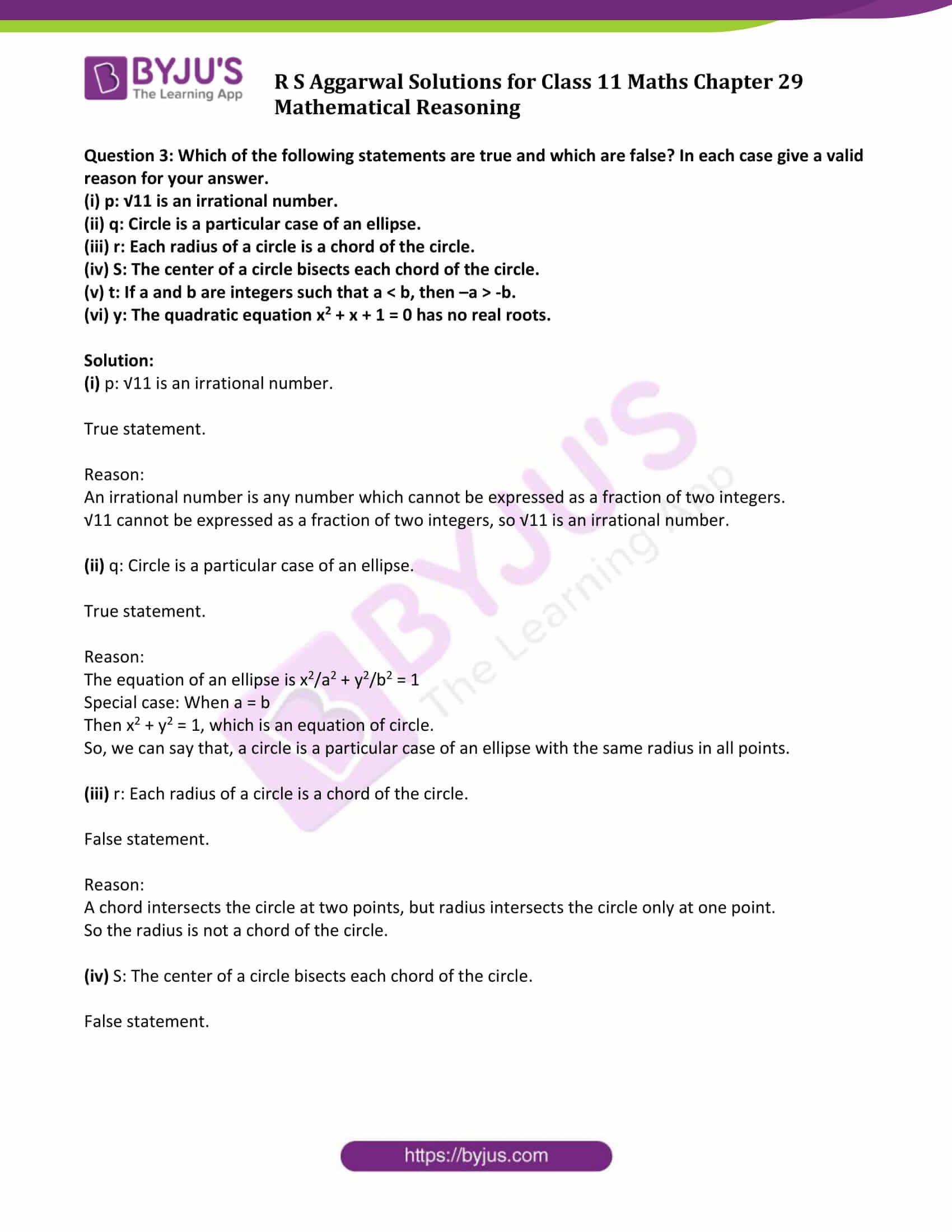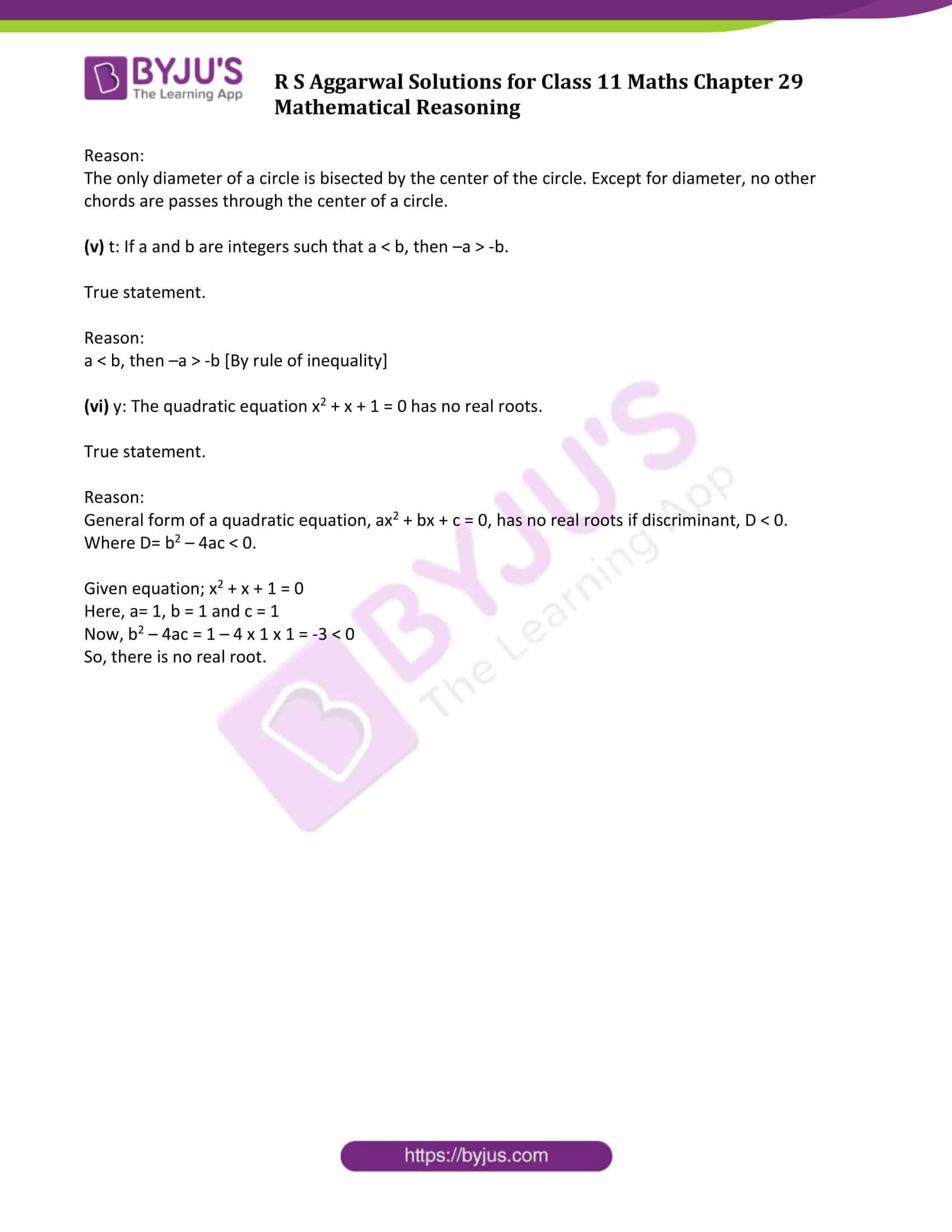### Access Answers to Maths R S Aggarwal Class 11 Chapter 29 Mathematical Reasoning Exercise 29A Page number 898

Question 1: Which of the following sentences are statements? In case of a statement mention whether it is true or false.

(i) The sun is a star.

(ii) √7 is an irrational number.

(iii) The sum of 5 and 6 is less than 10.

(v) Ice is always cold.

(vi) Have you ever seen the Red Fort?

(vii) Every relation is a function.

(viii) The sum of any two sides of a triangle is always greater than the third side.

(ix) May God bless you!

Solution:

(i) The sun is a star is a statement.

It is a scientifically proven fact, therefore this sentence is always true.

(ii) An irrational number is any number which cannot be expressed as a fraction of two integers.

Here, √7 cannot be expressed as a fraction of two integers, so √7 is an irrational number.

Therefore, “√7 is an irrational number” is a statement, and it is true.

(iii) Sum of 5 and 6 = 5 + 6 = 11 > 10

Sum of 5 and 6 is 11, which is greater than 10.

Therefore, “The sum of 5 and 6 is less than 10” is a statement, but not true.

(iv) The sentence ‘Go to your class’ is an order.

This is an Imperative sentence. Hence it is not a statement.

(v) Ice is always cold is a statement.

It is scientifically proven the fact, therefore the sentence is always true.

(vi) The sentence ‘Have you ever seen the Red Fort?

This is an interrogative sentence. Hence not a statement.

(vii) ‘Every relation is a function’ is a statement.

There are relations which are not functions.

Therefore, the sentence is false.

(viii) ‘The sum of any two sides of a triangle is always greater than the third side’

It is a statement and mathematically proven result.

Hence the statement is true.

(ix) ‘May God bless you!’ is an exclamation sentence. Hence it is not a statement.

Question 2: Which of the following sentences are statements? In case of a statement, mention whether it is true or false.

(i) Paris is in France.

(ii) Each prime number has exactly two factors.

(iii) The equation x2 + 5|x| + 6 = 0 has no real roots.

(iv) (2 + √3) is a complex number.

(v) Is 6 a positive integer?

(vi) The product of -3 and -2 is -6.

(vii) The angles opposite the equal sides of an isosceles triangle are equal.

(viii) Oh! it is too hot.

(ix) Monika is a beautiful girl.

(x) Every quadratic equation has at least one real root.

Solution:

(i) Paris is in France, is a statement.

Paris is located in France, so the sentence is true.

So, the statement is true.

(ii) Each prime number has exactly two factors, is a statement.

This is a mathematically proven fact.

So, the statement is true.

(iii) The equation x2 + 5|x| + 6 = 0 has no real roots.

Find the roots of x2 + 5|x| + 6 = 0:

Case 1: x ≥ 0

x2 + 5x + 6 = (x+2)(x+3) = 0 ⇒ x= −2, −3 but we already assumed x ≥ 0, which is a contradiction.

Case 2: x < 0

x2 − 5x + 6 = (x−2)(x−3) = 0 ⇒ x = (2,3) but we already assumed x < 0, which is a contradiction.

So, equation x2 + 5|x| + 6 = 0 has no real roots.

Therefore, the given sentence is true, and it is a statement.

(iv) (2 + √3) is a complex number, is a statement.

Complex numbers are in the form ‘a+ib’.

(2 + √3) cannot be expressed in ‘a+ib’ form,.

2 + √3 is not a complex number.

The given sentence is a statement, and it is false.

(v) Is 6 a positive integer?

This is an interrogative sentence, so it is not a statement.

(vi) The product of -3 and -2 is -6, is a statement.

Product of -3 and -2 = -3 x -2 = 6 ≠ -6

This statement is false.

(vii) The angles opposite the equal sides of an isosceles triangle are equal, is a statement.

It is a mathematically proven result.

So the given sentence is true.

(viii) Oh! it is too hot.

This is an exclamatory sentence, so it is not a statement.

(ix) Monika is a beautiful girl, is not a statement.

The given sentence is an opinion, can be true for some cases, false for some other case.

(x) Every quadratic equation has at least one real root, is a statement.

Because not every quadratic equation will have a real root.

So the given sentence is false.

Question 3: Which of the following statements are true and which are false? In each case give a valid reason for your answer.

(i) p: √11 is an irrational number.

(ii) q: Circle is a particular case of an ellipse.

(iii) r: Each radius of a circle is a chord of the circle.

(iv) S: The center of a circle bisects each chord of the circle.

(v) t: If a and b are integers such that a < b, then –a > -b.

(vi) y: The quadratic equation x2 + x + 1 = 0 has no real roots.

Solution:

(i) p: √11 is an irrational number.

True statement.

Reason:

An irrational number is any number which cannot be expressed as a fraction of two integers.

√11 cannot be expressed as a fraction of two integers, so √11 is an irrational number.

(ii) q: Circle is a particular case of an ellipse.

True statement.

Reason:

The equation of an ellipse is x2/a2 + y2/b2 = 1

Special case: When a = b

Then x2 + y2 = 1, which is an equation of circle.

So, we can say that, a circle is a particular case of an ellipse with the same radius in all points.

(iii) r: Each radius of a circle is a chord of the circle.

False statement.

Reason:

A chord intersects the circle at two points, but radius intersects the circle only at one point.

So the radius is not a chord of the circle.

(iv) S: The center of a circle bisects each chord of the circle.

False statement.

Reason:

The only diameter of a circle is bisected by the center of the circle. Except for diameter, no other chords are passes through the center of a circle.

(v) t: If a and b are integers such that a < b, then –a > -b.

True statement.

Reason:

a < b, then –a > -b [By rule of inequality]

(vi) y: The quadratic equation x2 + x + 1 = 0 has no real roots.

True statement.

Reason:

General form of a quadratic equation, ax2 + bx + c = 0, has no real roots if discriminant, D < 0.

Where D= b2 – 4ac < 0.

Given equation; x2 + x + 1 = 0

Here, a= 1, b = 1 and c = 1

Now, b2 – 4ac = 1 – 4 x 1 x 1 = -3 < 0

So, there is no real root.

## Access other exercise solutions of Class 11 Maths Chapter 29 Mathematical Reasoning

Exercise 29B Solutions

Exercise 29C Solutions

Exercise 29D Solutions

## R S Aggarwal Solutions for Class 11 Maths Chapter 29 Exercise 29A

Class 11 Maths Chapter 29 Mathematical Reasoning Exercise 29A is based on the following topics:

• Sentences

-Declarative sentences

-Imperative sentences

-Interrogative sentences and

-Exclamatory sentences

• Statements
• Negation of a statement